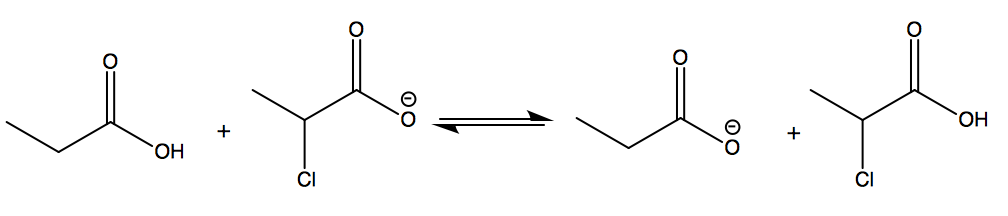Problem: Refer to the following equation to answer the questions below.  a. The strongest Bronsted-Lowry acid in the equation is _______. b. Will this reaction take place as written (from left to right)? Explain.

FREE Expert Solution
94% (338 ratings)
Problem Details

Refer to the following equation to answer the questions below.

a. The strongest Bronsted-Lowry acid in the equation is _______.

b. Will this reaction take place as written (from left to right)? Explain.What scientific concept do you need to know in order to solve this problem?

Our tutors have indicated that to solve this problem you will need to apply the Ranking Acidity concept. You can view video lessons to learn Ranking Acidity Or if you need more Ranking Acidity practice, you can also practice Ranking Acidity practice problems .

What is the difficulty of this problem?

Our tutors rated the difficulty of Refer to the following equation to answer the questions belo... as medium difficulty.

How long does this problem take to solve?

Our expert Organic tutor, Chris took 5 minutes to solve this problem. You can follow their steps in the video explanation above.

What professor is this problem relevant for?

Based on our data, we think this problem is relevant for Professor Kaden's class at UCF.